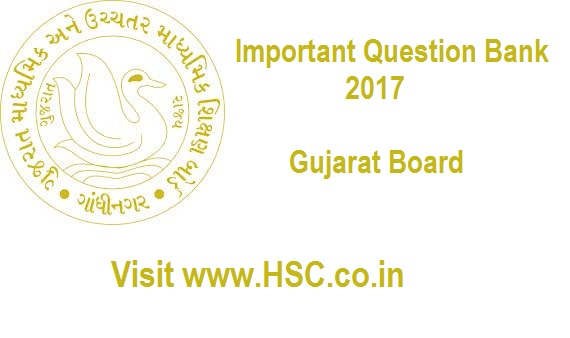# Math’s – Important Question Bank for Gujarat (12th Std) HSC Board Exam 2017

HSC Board Exams are fast approaching and students are getting anxious about how to prepare for their HSC Board Exams. So we had mentioned some HSC Study Tips to help students in Cracking HSC Exams.

After the tremendous success of our last year  we have also created a list of Most Important Question Bank for Gujarat (12th Std) HSC Board Exam 2017 which are likely to appear in HSC Board Exams this year.To unlock the content Click on any of 3 buttons available in the box below saying “This content is Locked”. Once you click on the button the content will get unlocked on same page itself. You must click on social media button showing in below box ie Facebook, Twitter or Google Plus to unlock the content.

Hi, we’re trying to collate and gather the data and would be updating it here a few days before the exam. Please keep on visiting our website for updates.

Please use the comments box below and post questions that you think are important from your analysis. It would help the HSC community a lot.

Do subscribe to our updates so that you do not miss out on any important information that we push your way.

1. Obtain the necessary and sufficient condition for A)z1 , y1), B(x2,y2) and C(x3,y3) to be collinear.
2. If the points (P+1,1), (2P+1, 3) and (2P + 2, 2P) are collinear then prove that p = 2 or p = -1/2.
3. Find the points which divide the line segments joining (1,2) & (2,1) into n – equal parts,
4. If A, B, C and P are distinct non collinear points of the plane, prove that area of ΔPAB + Area of ΔPBC + Area of Δ PCA ≥ Area of ΔABC.
5. Find the equation of the line passing through (1, 4) and making intercepts a and b on X and Y axes respectively. If the area of the triangle formed by the line and the co-ordinate exes in the first quadrant is 8 units.
6. Prove that the graph of a linear equation in R2 is a line.
7. If G is the centroid of Δ ABC then prove that

AB2 + BC2 + CA2 = 3(GA2 +GB2 +GC2).

1. An adjacent pair of vertices of a square is (-1,3) and (2, -1). Find the remaining vertices.
2. Prove that the points (3,4) and (-2,1) are on the opposite side of the line 3x – y + 6 =0
3. Obtain the Cartesian equation of a line passing through A(x1, y1), B(x2,y2)
4. Obtain length of a perpendicular segment from the origin to the line ax + by + C = 0, a2 + b2 = 0.
5. Obtain angel between two intersecting lines.
6. Define distance function in R2. State and prove the properties of distance function in R2
7. If a + b = ab then prove that the points (a. 0), (0,b) and (1,1) are collinear.
8. If P(2,7) divides the line segment joining A(-1,1) and B in the ration 3:2, find the co-ordinates of B.
9. Define the slope of a line. Obtain the formula for measure of angel between two intersecting lines.
10. Suppose that 3 out of 100 men and 3 out of 1000 women in a city suffer from color blindness. A random selected person of the city is found to be color blind. If a person is a man or woman is assumed to be equally likely, what is the probability that the selected person is a woman?
11. Distinguish between bit and byte
12. Covet (32.34)8 into binary numbers and decimal numbers.
13. Two faces of balanced die are marked with integer 2 and 3 and other sides are blank. If we toss balanced die five times then what the probability of getting sum of integers exactly 12?
14. The percentage of students passing in three subjects A B and C are show below A: 50%, B: 40% C: 30%, A and B : 35% B and C : 20%, A and C : 25%, A and B and C: 15%, find the percentage of students passing in at least one subject.
15. (a). State the names of electronic components used in the third and fourth generation of computers.

(B) If A1, A2 and A3 one mutually independent events then                     prove that A1’, A2 ᴖ A3

1. (a) Define (i) Equiprobable Primary events

(II) intersection events

(b) If A and B are independent events then prove that A and B’,            A’ and B and A’ and B’ are also indigents events.

1. (a) State and prove the rule of addition of probability

OR

(ii) Classical definition of probability

(b) state and prove Baye’s rule of probability

1. 13 cards are selected at random from a pack of 52 cards. Find the probability that 3 out of 13 cards are kings.
2. If E and F are independent events and if G denotes the event that only one out of events E and F occurs then show that –

P(G) = P(E) + P(F) – 2p(E), P(F)

27. Define Axiomatic definition of probability and pair wise independent events.

28. A box has 10 black and 4 white balls, if 3 balls are drawn at random from the box, what is the probability of the event that

(a) All are white?

(b) At least one is white?

(c) At most one is white?

29. Find probability of getting 5 Monday in month of April

30. i) State the main parts of computer.

ii) Write the binary form of the decimal number, (1000)10

iii) Subtract 0.4891 E -07 from 0.1645-05.

Don’t forget to read : MUST REMEMBER THINGS on the day of Exam for HSC Students

1.keyur says: